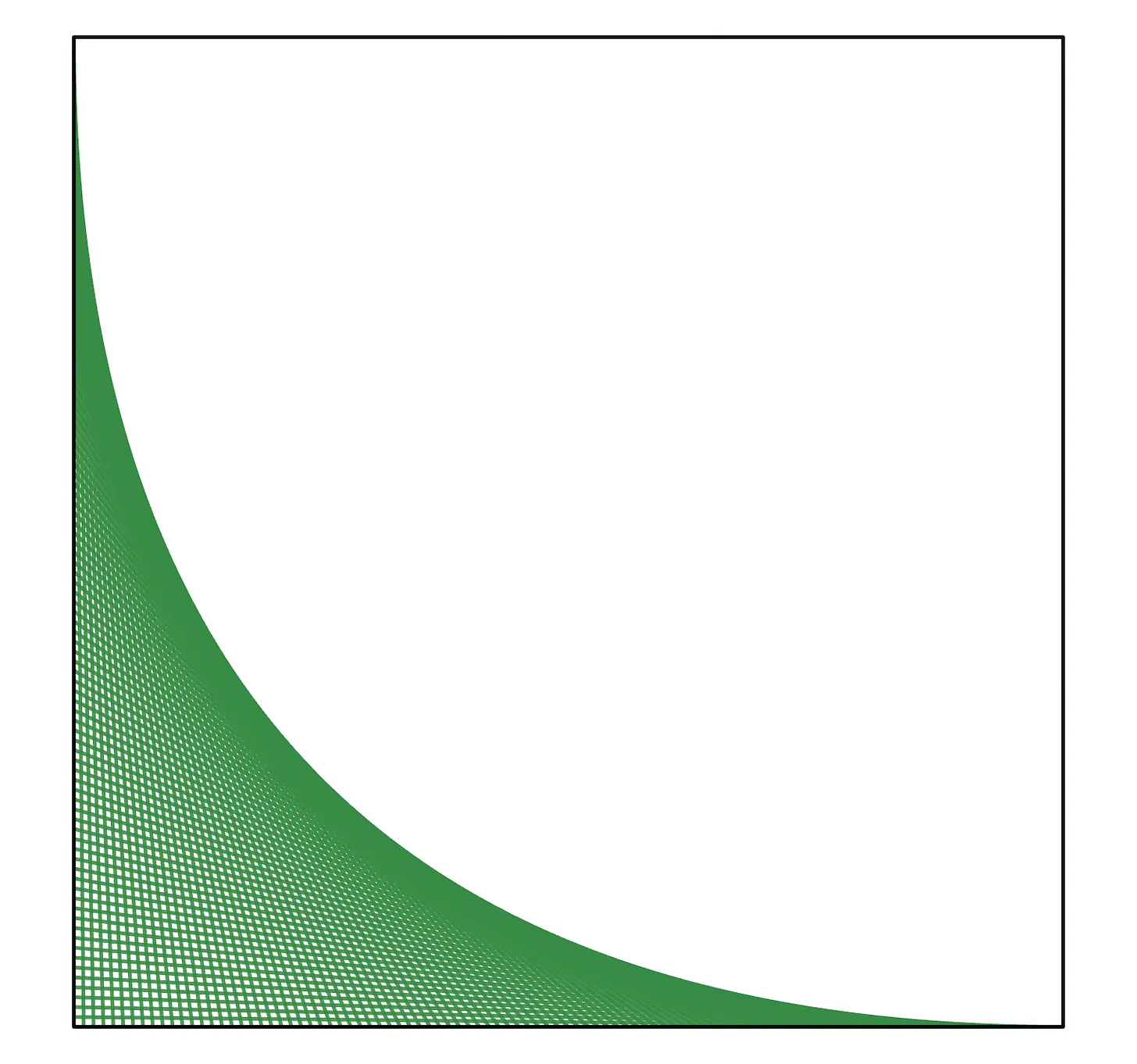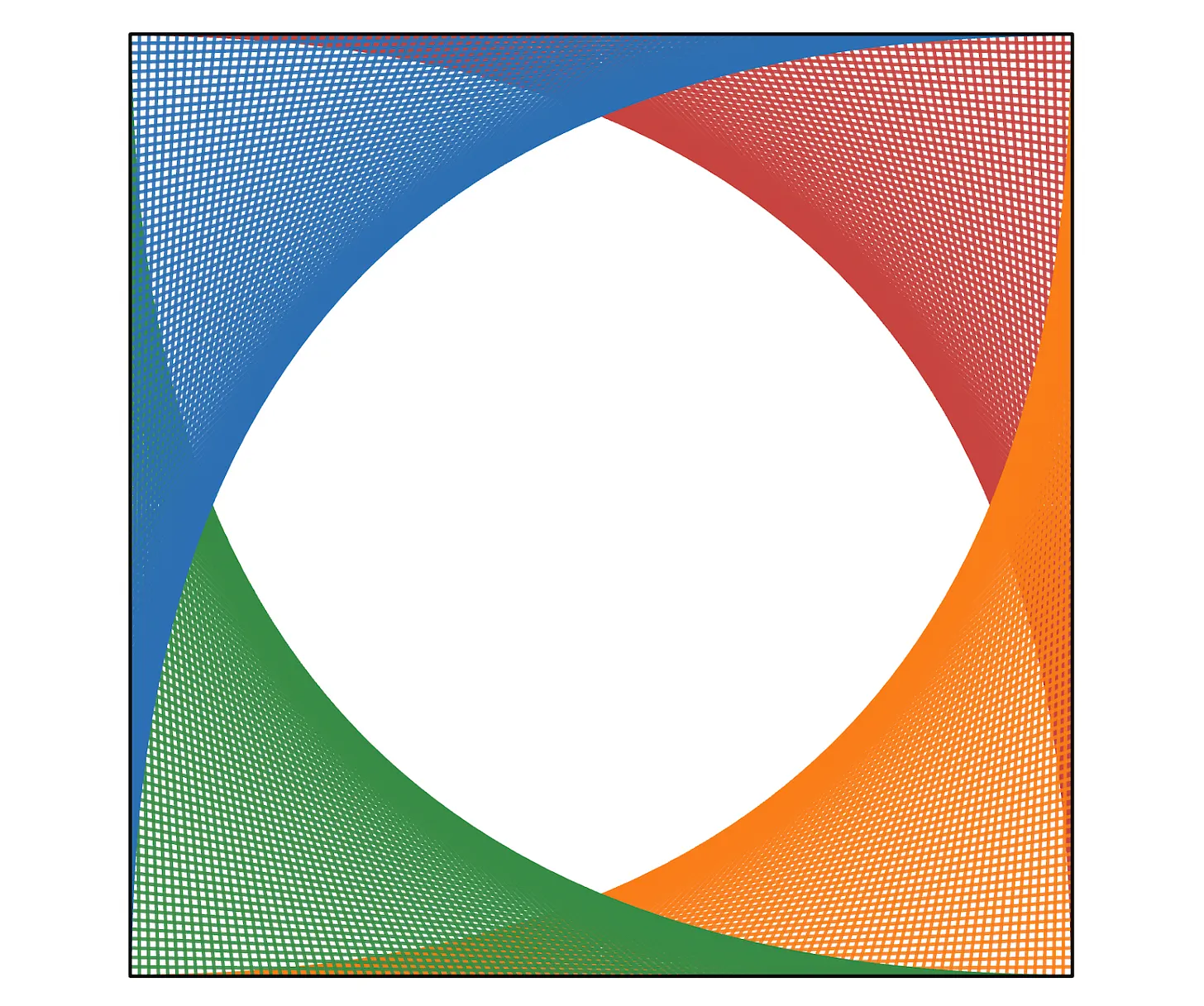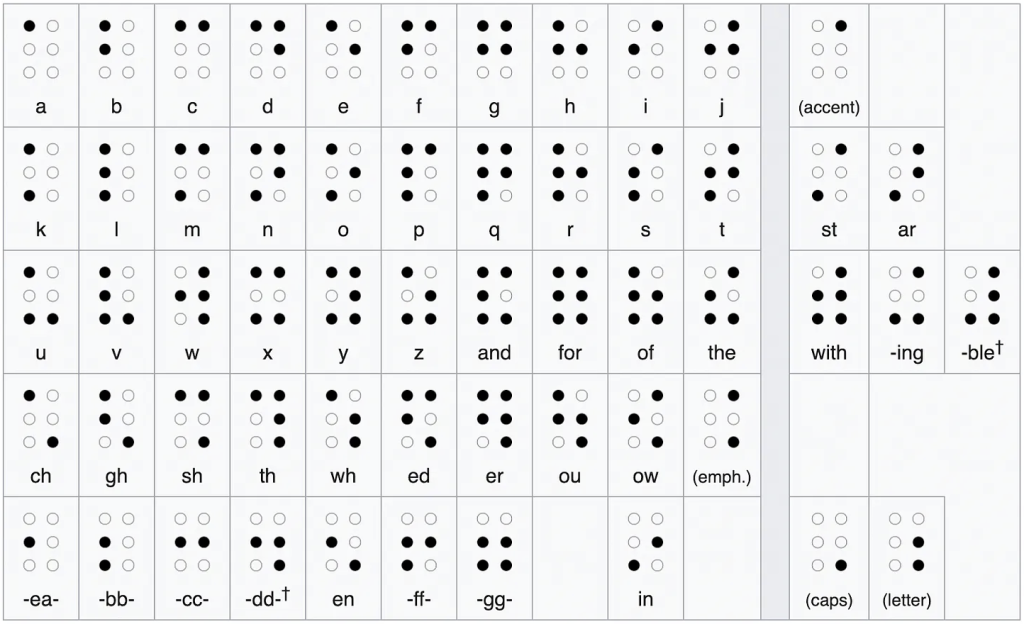## Pinball probability

This week’s Fiddler is a challenging probability question.

You’re playing a game of pinball that includes four lanes, each of which is initially unlit. Every time you flip the pinball, it passes through exactly one of the four lanes (chosen at random) and toggles that lane’s state. So if that lane is unlit, it becomes lit after the ball passes through. But if the lane is lit, it becomes unlit after the ball passes through. On average, how many times will you have to flip the pinball until all four lanes are lit?

Extra credit: Instead of four lanes, now suppose your pinball game has $n$ lanes. And let’s say that $E(n)$ represents the average number of pinball flips it takes until all $n$ lanes are lit up. Now, each time you increase the number of lanes by one, you find that it takes you approximately twice as long to light up all the lanes. In other words, $E(n+1)$ seems to be about double $E(n)$. But upon closer examination, you find that it’s not quite double. Moreover, there’s a particular value of $n$ where the ratio $E(n+1)/E(n)$ is at a minimum. What is this value of $n$?

My solution:
[Show Solution]

## The weaving loom problem

This week’s Fiddler is a classic problem.

A weaving loom consists of equally spaced hooks along the x and y axes. A string connects the farthest hook on the x-axis to the nearest hook on the y-axis, and continues back and forth between the axes, always taking up the next available hook. This leads to a picture that looks like this:As the number of hooks goes to infinity, what does the shape trace out?

Extra credit: If four looms are rotated and superimposed as shown below, what is the area of the white region in the middle?My solution:
[Show Solution]

## Betting on football with future knowledge

This week’s Fiddler is a football-themed puzzle with a twist: you can see the future! Sort of.

You know ahead of time that your football team will win 8 of their 12 remaining games, but you don’t know which ones. You can place bets on every game, placing bets either for or against your team. You can bet any amount up to how much you currently have. You want to implement a betting strategy that guarantees you’ll have as much money as possible after the 12 games are complete. If you did so, then after the 12 games how much money would you be guaranteed to have if you started with $100? My solution: [Show Solution] A more elegant alternative solution, due in large part to a clever observation by Vince Vatter. [Show Solution] ## Optimal baseball lineup This week’s Fiddler is a problem about how to set the optimal baseball lineup. Eight of your nine batters are “pure contact” hitters. One-third of the time, each of them gets a single, advancing any runners already on base by exactly one base. (The only way to score is with a single with a runner on 3rd). The other two-thirds of the time, they record an out, and no runners advance to the next base. Your ninth batter is the slugger. One-tenth of the time, he hits a home run. But the remaining nine-tenths of the time, he strikes out. Your goal is to score as many runs as possible, on average, in the first inning. Where in your lineup (first, second, third, etc.) should you place your home run slugger? Extra Credit: Instead of scoring as many runs as possible in the first inning, you now want to score as many runs as possible over the course of nine innings. What’s more, instead of just having one home run slugger, you now have two sluggers in your lineup. The other seven batters remain pure contact hitters. Where in the lineup should you place your two sluggers to maximize the average number of runs scored over nine innings? My solution: [Show Solution] ## Braille puzzle This week’s Fiddler is a counting problem about the Braille system. Braille characters are formed by raised dots arranged in a braille cell, a three-by-two array. With six locations for dots, each of which is raised or unraised, there are 26, or 64, potential braille characters. Each of the 26 letters of the basic Latin alphabet (shown below) has its own distinct arrangement of dots in the braille cell. What’s more, while some arrangements of raised dots are rotations or reflections of each other (e.g., E and I, R and W, etc.), no two letters are translations of each other. For example, only one letter (A) consists of a single dot – if there were a second such letter, A and this letter would be translations of each other.Of the 64 total potential braille characters, how many are in the largest set such that no two characters consist of raised dot patterns that are translations of each other? Extra Credit In addition to six-dot braille, there’s also an eight-dot version. But what if there were even more dots? Let’s quadruple the challenge by doubling the size of the cell in each dimension. Consider a six-by-four array, where a raised dot could appear at each location in the array. Of the 224 total potential characters, how many are in the largest set such that no two characters are translations of each other? My solution: [Show Solution] ## Making something out of nothing This week’s Fiddler is a problem about composing functions. Here it goes: Consider$f(n) = 2n+1$and$g(n) = 4n$. It’s possible to produce different whole numbers by applying combinations of$f$and$g$to$0$. How many whole numbers between$1$and$1024$(including$1$and$1024$) can you produce by applying some combination of$f$’s and$g$’s to the number$0$? Extra Credit: Now consider the functions$g(n) = 4n$and$h(n) = 1−2n$. How many integers between$-1024$and$1024$(including$-1024$and$1024$) can you produce by applying some combination of$g$’s and$h$’s to the number$0? My solution: [Show Solution] ## Möbius prism This week’s Fiddler is a problem about a multi-sided Möbius prism: Consider an three-dimensional prism whose bases are regular N-gons. I twist it and stretch it into a loop, before finally connecting the two bases. Suppose that my twist is by a random angle, such that the two bases are aligned when they are coonected. Among all whole number values of N less than or equal to 1,000, for which value of N will a randomly twisted regular N-gon prism have the most distinct faces, on average? My solution: [Show Solution] ## How much can you pull out of a hat? This week’s Riddler Classic is a strategy game about maximizing payout. What is the optimal strategy? You start with just the number 1 written on a slip of paper in a hat. Initially, there are no other slips of paper in the hat. You will draw from the hat 100 times, and each time you draw, you have a choice: If the number on the slip of paper you draw is k, then you can either receive k dollars or add k higher numbers to the hat. For example, if the hat were to contain slips with the numbers 1 through 6 and you drew a 4, you could either receive4 or receive no money but add four more slips numbered 7, 8, 9 and 10 into the hat. In either case, the slip with the number 4 would then be returned to the hat.

If you play this game perfectly — that is, to maximize the total amount of money you’ll receive after all 100 rounds — how much money would you expect to receive on average?

My solution:
[Show Solution]

## Making the fastest track

This week’s Riddler Classic is a problem about minimum-time optimization.

While passing the time at home one evening, you decide to set up a marble race course. No Teflon is spared, resulting in a track that is effectively frictionless. The start and end of the track are 1 meter apart, and both positions are 10 centimeters off the floor. It’s up to you to design a speedy track. But the track must always be at floor level or higher — please don’t dig a tunnel through your floorboards. What’s the fastest track you can design, and how long will it take the marble to complete the course?

My solution:
[Show Solution]

## Ellipse packing

You’ve heard of circle packing… Well this week’s Riddler Classic is about ellipse packing!

This week, try packing three ellipses with a major axis of length 2 and a minor axis of length 1 into a larger ellipse with the same aspect ratio. What is the smallest such larger ellipse you can find? Specifically, how long is its major axis?

Extra credit: Instead of three smaller ellipses, what about other numbers of ellipses?

My solution:
[Show Solution]